# Mathematics - Signals and Systems - Exercises on the Z Transform

in StemSocial3 months ago[Image1]

## Introduction

Hey it's a me again @drifter1!

Today we continue with my mathematics series about Signals and Systems to get into Exercises on the Z Transform.

So, without further ado, let's dive straight into it!

## Z Transform and ROC Examples [Based on 22.4 from Ref1]

Let's determine the Z Transform, pole-zero plot and associated ROC for each of the following cases: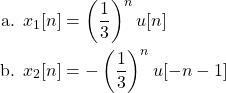### a.

The signal x1[n] is a common case, which is included in Z Transform tables. As such, it has a well-known Z Transform and ROC, which is given by: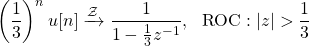The root of the denominator is a pole, and at point 1/3 in the real-axis. So, at last, the pole-zero plot and ROC can be visualized as follows: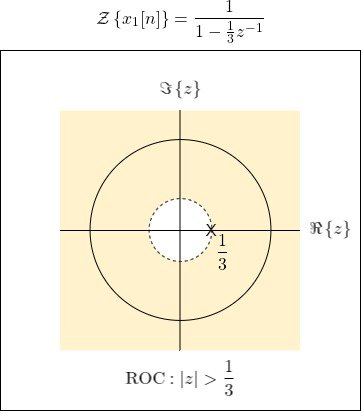### b.

The second signal, x2[n] has the same exact algebraic expression for the Z Transform. The only difference is the ROC, which is now the inner circle. So, the Z Transform and ROC are as follows: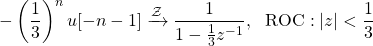And visually we have: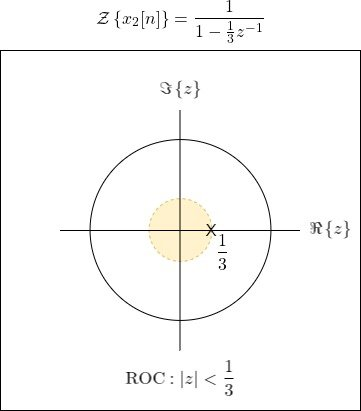## ROC from Conditions [Based on 22.3 from Ref1]

Let's consider the following pole-zero plot of the Z Transform X(z) of a sequence x[n]: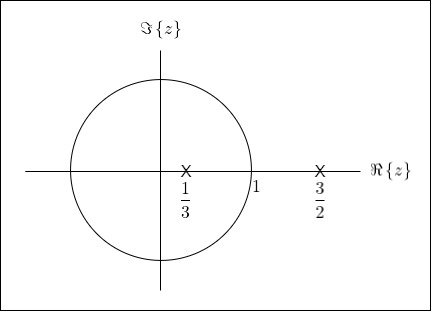Determine the associated region of convergence (ROC) for each of the following conditions:

1. x[n] is right-sided
2. x[n] is left-sided
3. The Fourier transform of x[n] converges
4. The Fourier transform of x[n] doesn't converge

### 1.

For a signal to be right-sided, the ROC must be of the form |z| > a. And, since the ROC can't include poles the ROC is: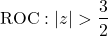### 2.

Now, for it to be left-sided, it's ROC must be of the form |z| < a, and not include poles, which leads to: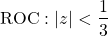### 3.

In order to converge, the ROC must include the unit circle (|z| = 1). And, because it must be bounded by poles, the ROC can only be: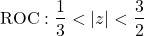### 4.

For the FT of x[n] to not converge, the ROC must not contain the unit circle, which means that there are two possibilities: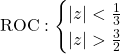## LTI System Analysis Example

Consider a discrete-time LTI system, with the following transfer function: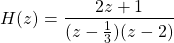Let's specify and sketch the ROC (and pole-zero plot) for each of the following cases:

1. The system is causal
2. The system is stable

### Poles and Zeros

First of all, in any of these cases the corresponding poles and zeros are as follows:

• The root of the numerator polynomial z = - 1/2 is a zero.
• The roots of the denominator polynomial z = 1/3 and z = 2 are the poles.

This leads to the following pole-zero diagram, which will be shared amongst the follow-up cases: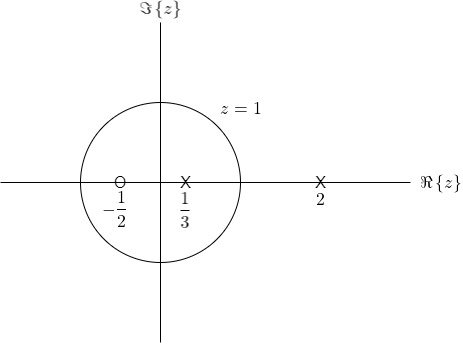### 1. Causal

As we know, a system is causal when the ROC is the outside region of a circle. In the case of rational functions it must also be:

• outside of the most distant pole, and
• the degree of the numerator must not be larger then the degree of the denominator polynomial

So, in the case of this system, the ROC is: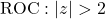or graphically: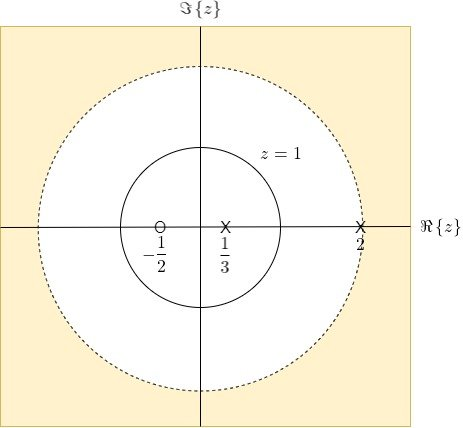### 2. Stable

For a system to be stable, the ROC must simply contain the unit circle.

As such, the ROC can be either of the following: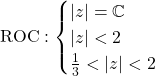and so either the complete z-region, the region below the circle at 2 or the intermediate region of two circles (one at 1/3 and one at 2).

Graphically: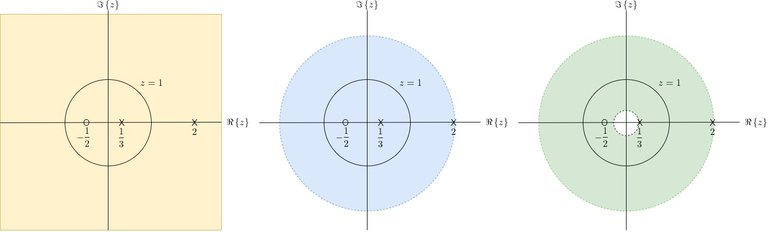`Let's note that it's impossible for this system to be both causal and stable!`

## RESOURCES:

### Images

Block diagrams and other visualizations were made using draw.io

## Previous articles of the series

### Laplace and Z Transforms

• Laplace Transform → Laplace Transform, Region of Convergence (ROC)
• Laplace Transform Properties → Linearity, Time- and Frequency-Shifting, Time-Scaling, Complex Conjugation, Multiplication and Convolution, Differentation in Time- and Frequency-Domain, Integration in Time-Domain, Initial and Final Value Theorems
• LTI System Analysis using Laplace Transform → System Properties (Causality, Stability) and ROC, LCCDE Representation and Laplace Transform, First-Order and Second-Order System Analysis
• Exercises on the Laplace Transform → Laplace Transform and ROC Examples, LTI System Analysis Example
• Z Transform → Z Transform, Region of Convergence (ROC), Inverse Z Transform
• Z Transform Properties → Linearity, Time-Shifting, Time-Scaling, Time-Reversal, z-Domain Scaling, Conjugation, Convolution, Differentation in the z-Domain, Initial and Final value Theorems
• LTI System Analysis using Z Transform → System Properties (Causality, Stability), LCCDE Representation and Z Transform

## Final words | Next up

And this is actually it for today's post!

From next time on, we will start getting into other various topics related to the Laplace and Z Transform...

See Ya!Keep on drifting!